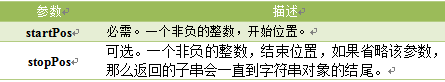javascript技巧# 详解JavaScript的内置对象

JavaScript 中的所有事物都是对象，如:字符串、数值、数组、函数等，每个对象带有属性和方法。

JavaScript 提供多个内建对象，比如 String、Date、Array 等等，使用对象前先定义，如下使用数组对象：

var objectName =new Array();//使用new关键字定义对象

`var objectName =[];`

`objectName.propertyName`

```var myarray=new Array(6);//定义数组对象
var myl=myarray.length;//访问数组长度length属性```

`objectName.methodName()`

```var mystr="Hello world!";//创建一个字符串
var request=mystr.toUpperCase(); //使用字符串对象方法```

Date 日期对象

`var Udate=new Date();`

```var d = new Date(2012, 10, 1); //2012年10月1日
var d = new Date('Oct 1, 2012'); //2012年10月1日```

Date对象中处理时间和日期的常用方法：get/setFullYear() 返回/设置年份，用四位数表示。

```var mydate=new Date();//当前时间2014年3月6日
document.write(mydate+"<br>");//输出当前时间
document.write(mydate.getFullYear()+"<br>");//输出当前年份
mydate.setFullYear(81); //设置年份
document.write(mydate+"<br>"); //输出年份被设定为 0081年。```

```Thu Mar 06 2014 10:57:47 GMT+0800
2014
Thu Mar 06 0081 10:57:47 GMT+0800```

• 结果格式依次为：星期、月、日、年、时、分、秒、时区。(火狐浏览器)
• 不同浏览器，时间格式有差异。

getDay() 返回星期，返回的是0-6的数字，0 表示星期天。如果要返回相对应“星期”，通过数组完成，代码如下:

```<script type="text/javascript">
var mydate=new Date();//定义日期对象
var weekday=["星期日","星期一","星期二","星期三","星期四","星期五","星期六"];
//定义数组对象,给每个数组项赋值
var mynum=mydate.getDay();//返回值存储在变量mynum中
document.write(mydate.getDay());//输出getDay()获取值
document.write("今天是："+ weekday[mynum]);//输出星期几
</script>```

```3

```

get/setTime() 返回/设置时间，单位毫秒数，计算从 1970 年 1 月 1 日零时到日期对象所指的日期的毫秒数。

```<script type="text/javascript">
var mydate=new Date();
document.write("当前时间："+mydate+"<br>");
mydate.setTime(mydate.getTime() + 60 * 60 * 1000);
document.write("推迟一小时时间：" + mydate);
</script>```

• 一小时 60 分，一分 60 秒，一秒 1000 毫秒
• 时间推迟 1 小时,就是: “x.setTime(x.getTime() + 60 60 1000);”

String 字符串对象

`var mystr = "I love JavaScript!"`

stringObject.length; 返回该字符串的长度。

```var mystr="Hello World!";
var myl=mystr.length;```

```var mystr="Hello world!";
var mynum=mystr.toUpperCase();```

charAt() 方法可返回指定位置的字符。返回的字符是长度为 1 的字符串。

`stringObject.charAt(index)`1.字符串中第一个字符的下标是 0。最后一个字符的下标为字符串长度减一（string.length-1）。

2.如果参数 index 不在 0 与 string.length-1 之间，该方法将返回一个空字符串。

```<script type="text/javascript">
var mystr="I love JavaScript!"
document.write(mystr.charAt(2));
</script>```

`l`

indexOf() 方法可返回某个指定的字符串值在字符串中首次出现的位置。

`stringObject.indexOf(substring, startpos)`1.该方法将从头到尾地检索字符串 stringObject，看它是否含有子串 substring。

2.可选参数，从stringObject的startpos位置开始查找substring，如果没有此参数将从stringObject的开始位置查找。

3.如果找到一个 substring，则返回 substring 的第一次出现的位置。stringObject 中的字符位置是从 0 开始的。

1.indexOf() 方法区分大小写。

2.如果要检索的字符串值没有出现，则该方法返回 -1。

```<script type="text/javascript">
var str="I love JavaScript!"
document.write(str.indexOf("I") + "<br />");
document.write(str.indexOf("v") + "<br />");
document.write(str.indexOf("v",8));
</script>```

```0
4
9```

split() 方法将字符串分割为字符串数组，并返回此数组。

`stringObject.split(separator,limit)````var mystr = "www.baidu.com";
document.write(mystr.split(".")+"<br>");
document.write(mystr.split(".", 2)+"<br>");```

```www,baidu,com
www,baidu```

```document.write(mystr.split("")+"<br>");
document.write(mystr.split("", 5));```

```w,w,w,.,b,a,i,d,u,.,c,o,m
w,w,w,.,b```

substring() 方法用于提取字符串中介于两个指定下标之间的字符。

`stringObject.substring(starPos,stopPos)````<script type="text/javascript">
var mystr="I love JavaScript";
document.write(mystr.substring(7));
document.write(mystr.substring(2,6));
</script>```

```JavaScript
love```

substr() 方法从字符串中提取从 startPos位置开始的指定数目的字符串。

`stringObject.substr(startPos,length)````<script type="text/javascript">
var mystr="I love JavaScript!";
document.write(mystr.substr(7));
document.write(mystr.substr(2,4));
</script>```

```JavaScript!
love```

Math对象

Math对象，提供对数据的数学计算。

```<script type="text/javascript">
var mypi=Math.PI;
var myabs=Math.abs(-15);
document.write(mypi);
document.write(myabs);
</script>```

```3.141592653589793
15```

Math 对象属性Math 对象方法ceil() 方法可对一个数进行向上取整。

`Math.ceil(x)````<script type="text/javascript">
document.write(Math.ceil(0.8) + "<br />")
document.write(Math.ceil(6.3) + "<br />")
document.write(Math.ceil(5) + "<br />")
document.write(Math.ceil(3.5) + "<br />")
document.write(Math.ceil(-5.1) + "<br />")
document.write(Math.ceil(-5.9))
</script>```

```1
7
5
4
-5
-5```

floor() 方法可对一个数进行向下取整。

`Math.floor(x)````<script type="text/javascript">
document.write(Math.floor(0.8)+ "<br>")
document.write(Math.floor(6.3)+ "<br>")
document.write(Math.floor(5)+ "<br>")
document.write(Math.floor(3.5)+ "<br>")
document.write(Math.floor(-5.1)+ "<br>")
document.write(Math.floor(-5.9))
</script>```

```0
6
5
3
-6
-6```

round() 方法可把一个数字四舍五入为最接近的整数。

`Math.round(x)````<script type="text/javascript">
document.write(Math.round(1.6)+ "<br>");
document.write(Math.round(2.5)+ "<br>");
document.write(Math.round(0.49)+ "<br>");
document.write(Math.round(-6.4)+ "<br>");
document.write(Math.round(-6.6));
</script>```

```2
3
0
-6
-7```

random() 方法可返回介于 0 ~ 1（大于或等于 0 但小于 1 )之间的一个随机数。

`Math.random();`

```<script type="text/javascript">
document.write(Math.random());
</script>```

`0.190305486195328`

```<script type="text/javascript">
document.write((Math.random())*10);
</script>```

`8.72153625893887`

Array 数组对象

1.定义了一个空数组:

`var  数组名= new Array();`

2.定义时指定有n个空元素的数组：

`var 数组名 =new Array(n);`

3.定义数组的时候，直接初始化数据：

`var  数组名 = [<元素1>, <元素2>, <元素3>...];`

`var myArray = [2, 8, 6];`

length 用法：<数组对象>.length；返回：数组的长度，即数组里有多少个元素。它等于数组里最后一个元素的下标加一。concat() 方法用于连接两个或多个数组。此方法返回一个新数组，不改变原来的数组。

`arrayObject.concat(array1,array2,...,arrayN)````<script type="text/javascript">
var mya = new Array(3);
mya = "1";
mya = "2";
mya = "3";
document.write(mya.concat(4,5)+"<br>");
document.write(mya);
</script>```

```1,2,3,4,5
1,2,3```

```<script type="text/javascript">
var mya1= new Array("hello!")
var mya2= new Array("I","love");
var mya3= new Array("JavaScript","!");
var mya4=mya1.concat(mya2,mya3);
document.write(mya4);
</script>```

`hello!,I,love,JavaScript,!`

join()方法用于把数组中的所有元素放入一个字符串。元素是通过指定的分隔符进行分隔的。

`arrayObject.join(分隔符)````<script type="text/javascript">
var myarr = new Array(3);
myarr = "I";
myarr = "love";
myarr = "JavaScript";
document.write(myarr.join());
</script>```

`I,love,JavaScript`

```<sript type="text/javascript">
var myarr = new Array(3)
myarr = "I";
myarr = "love";
myarr = "JavaScript";
document.write(myarr.join("."));
</script>```

`I.love.JavaScript`

reverse() 方法用于颠倒数组中元素的顺序。

`arrayObject.reverse()`

```<script type="text/javascript">
var myarr = new Array(3)
myarr = "1"
myarr = "2"
myarr = "3"
document.write(myarr + "<br />")
document.write(myarr.reverse())
</script>```

```1,2,3
3,2,1```

slice() 方法可从已有的数组中返回选定的元素。

`arrayObject.slice(start,end)`1.返回一个新的数组，包含从 start 到 end （不包括该元素）的 arrayObject 中的元素。

2.如果 end 未被规定，那么 slice() 方法会选取从 start 到数组结尾的所有元素。

String.slice()与 Array.slice() 相似。

```<script type="text/javascript">
var myarr = new Array(1,2,3,4,5,6);
document.write(myarr + "<br>");
document.write(myarr.slice(2,4) + "<br>");
document.write(myarr);
</script>```

```1,2,3,4,5,6
3,4
1,2,3,4,5,6```

sort()方法使数组中的元素按照一定的顺序排列。

`arrayObject.sort(方法函数)`1.如果不指定<方法函数>，则按unicode码顺序排列。

2.如果指定<方法函数>，则按<方法函数>所指定的排序方法排序。

`myArray.sort(sortMethod);`

1.使用sort()将数组进行排序，代码如下：

```<script type="text/javascript">
var myarr1 = new Array("Hello","John","love","JavaScript");
var myarr2 = new Array("80","16","50","6","100","1");
document.write(myarr1.sort()+"<br>");
document.write(myarr2.sort());
</script>```

```Hello,JavaScript,John,love
1,100,16,50,6,80```

2.如要实现这一点，就必须使用一个排序函数，代码如下：

```<script type="text/javascript">
function sortNum(a,b) {
return a - b;
//升序，如降序，把“a - b”该成“b - a”
}
var myarr = new Array("80","16","50","6","100","1");
document.write(myarr + "<br>");
document.write(myarr.sort(sortNum));
</script>```

```80,16,50,6,100,1
1,6,16,50,80,100```